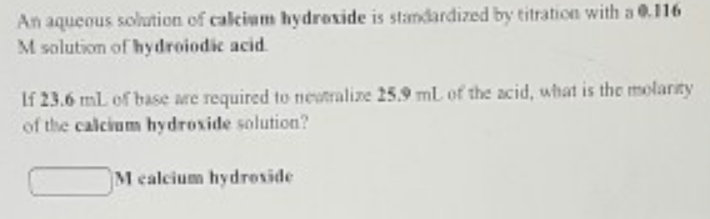# Problem: An aqueous solution of  calcium hydroxide is standardized by titration with a  0.116 M solution of hydroiodic acid.If 23.6 mL of base are required to  25.9 mL of the acid, what is the molarity of the  calcium hydroxide solution?

###### FREE Expert Solution
79% (79 ratings)###### Problem Details

An aqueous solution of  calcium hydroxide is standardized by titration with a  0.116 M solution of hydroiodic acid.

If 23.6 mL of base are required to  25.9 mL of the acid, what is the molarity of the  calcium hydroxide solution?